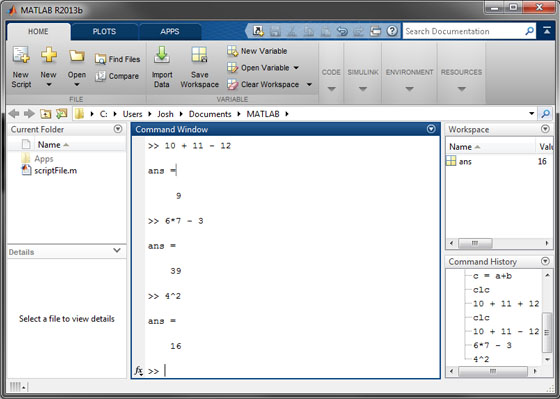# MATLAB as a Calculator

The most basic use of MATLAB is to use it as a fancy MATLAB calculator. This simply means we can execute mathematical operations in the command window much like you would with a handheld calculator.

To do this, we enter an expression, 10 + 11 - 2, at the command prompt and hit enter on the keyboard. We see that MATLAB immediately returns the the answers. We can continue to enter more expressions and MATLAB will continue to return answers:### Inline expression input

More complex expressions must be entered into the command prompt in inline format. In order to evaluate the expression  we would input

2.3^2/(6.9-3)

into the command prompt. For those who are new to computer programming, learning to input expressions correctly in the inline format can be difficult and will be the source of many frustrating MATLAB errors. I promise this will become much easier the more you practice!

### Operator precedence

MATLAB also uses a standard precedence for arithmetic commands that is identical to the order-of-operations used in algebra. This precedence determines the order in which commands are executed:

1. Parentheses () (do not use square [] or curly {} braces)
2. Exponents ^
3. Multiplication * and Division /
4. Addition + and Subtraction -

In addition, MATLAB always works from left to right, including commands that have equal precedence. The sign for both entering a negative number and performing subtraction is the same. Finally, one of the common mistakes I see for beginning programmers is forgetting that there are no implied operations in MATLAB. For example, you cannot multiply 10 by 2 by entering 10(2). You must enter either 10*(2) or simply 10*2.

### Storing data in variables

A useful feature in MATLAB (and in all computer programming languages for that matter) is the ability to store and recall data using variables. To do this in the MATLAB command window, simply type in a variable name followed by the equals sign and the number or expression whose result you wish to store. The stored value can now be retrieved (even for use in another expression) by simply using its name. Here it is important to note that assigning a new value to an existing variable will overwrite its existing value (which is sometimes desired). MATLAB variable names must begin with a letter and can contain upper/lower case letters, numbers, and underscores (_).

The picture below demonstrates some basic usage of variables in the command window. Notice that the value corresponding the variables can be seen in the workspace pane?### Built-in math functions

MATLAB has a number of built-in mathematical functions. These functions can be called by entering the function name immediately followed by the argument/input surrounded by parenthesis (for example functionName(inputValue)). Some common math functions available in MATLAB are given in the table below.

Function Description
sqrt() Square root
exp() Exponential function
log() Natural logarithm (commonly written as ln)
log10() Log base 10
sin() Value of sine with input given in radians
sind() Value of sine with input given in degrees
asin() Inverse sine of an angle in radians
asind() Inverse sine of an angle in degrees

In addition, some built-in functions do not take any input, but rather act like built-in constants. For example, entering pi returns the value of  to many decimal places of accuracy. In addition, the constants i and j return an imaginary number, which can also be obtained by entering sqrt(-1).

You can quickly obtain help for any built-in MATLAB function by entering help functionName at the command prompt. Try this to see what MATLAB has to say about the <asind function.

### Retrieving previous input

By pressing the up arrow key on the keyboard you can retrieve expressions or commands that have been previously entered in the command window. This is useful if you find an error in a long expression. You can simply cycle back to the expression and make the correction without retyping it.

Lets now move onto the next part of the tutorial where we will learn to program using MATLAB script files: Using MATLAB Script Files.

icon-long-arrow-left Last icon-cog Next icon-long-arrow-right